## ML Aggarwal Class 10 Solutions for ICSE Maths Chapter 7 Factorization Chapter Test

These Solutions are part of ML Aggarwal Class 10 Solutions for ICSE Maths. Here we have given ML Aggarwal Class 10 Solutions for ICSE Maths Chapter 7 Factorization Chapter Test.

Question 1.
Find the remainder when 2x3 – 3x2 + 4x + 7 is divided by
(i) x – 2
(ii) x + 3
(iii) 2x + 1
Solution:
f(x) = 2x3 – 3x2 + 4x + 7
(i) Let x – 2 = 0, then x = 2
Substituting value of x in f(x)
f(2) = 2 (2)3 – 3 (2)2 + 4 (2) + 7
= 2 × 8 – 3 × 4 + 4 × 2 + 7
= 16 – 12 + 8 + 7 = 19
Remainder = 19 Ans.
(ii) Let x + 3 = 0, then x = – 3
Substituting the value of x in f(x)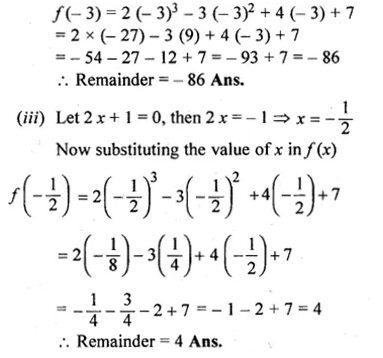Question 2.
When 2x3 – 9x2 + 10x – p is divided by (x + 1), the remainder is – 24.Find the value of p.
Solution:
Let x + 1 = 0 then x = – 1,
Substituting the value of x in f(x)
f(x) = 2x3 – 9x2 + 10x – pQuestion 3.
If (2x – 3) is a factor of 6x2 + x + a, find the value of a. With this value of a, factorise the given expression.
Solution:
Let 2 x – 3 = 0 then 2x = 3
=>x = $$\\ \frac { 3 }{ 2 }$$
Substituting the value of x in f(x)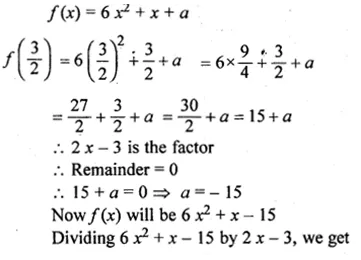Question 4.
When 3x2 – 5x + p is divided by (x – 2), the remainder is 3. Find the value of p. Also factorise the polynomial 3x2 – 5x + p – 3.
Solution:
f(x) = 3x2 – 5x+ p
Let (x – 2) = 0, then x = 2
f(2) = 3 (2)2 – 5(2) + p
= 3 x 4 – 10 + p
= 12 – 10 + p
= 2 + pQuestion 5.
Prove that (5x + 4) is a factor of 5x3 + 4x2 – 5x – 4. Hence factorise the given polynomial completely.
Solution:
f(x) = 5x3 + 4x2 – 5x – 4
Let 5x + 4 = 0, then 5x = – 4
=> x = $$\\ \frac { -4 }{ 2 }$$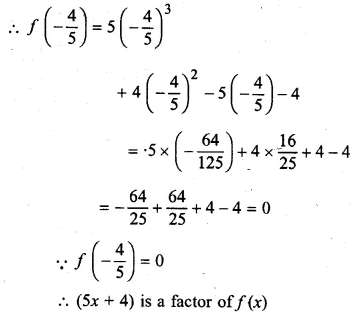Question 6.
Use factor theorem to factorise the following polynomials completely:
(i) 4x3 + 4x2 – 9x – 9
(ii) x3 – 19x – 30
Solution:
(i) f(x) = 4x3 + 4x2 – 9x – 9
Let x = – 1,then
f( – 1) = 4 ( – 1)3 + 4 ( – 1)2 – 9 ( – 1) – 9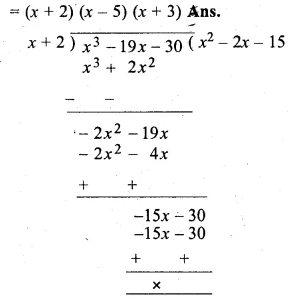Question 7.
If x3 – 2x2 + px + q has a factor (x + 2) and leaves a remainder 9, when divided by (x + 1), find the values of p and q. With these values of p and q, factorise the given polynomial completely.
Solution:
f(x) = x3 – 2x2 + px + q
(x + 2) is a factor
f( – 2) = ( – 2)3 – 2( – 2)2 + p ( – 2) + q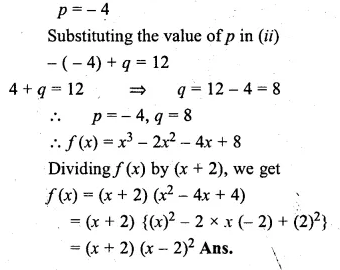Question 8.
If (x + 3) and (x – 4) are factors of x3 + ax2 – bx + 24, find the values of a and b: With these values of a and b, factorise the given expression.
Solution:
f(x) = x3 + ax2 – bx + 24
Let x + 3 = 0, then x = – 3
Substituting the value of x in f(x)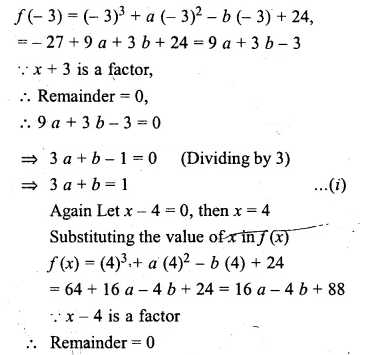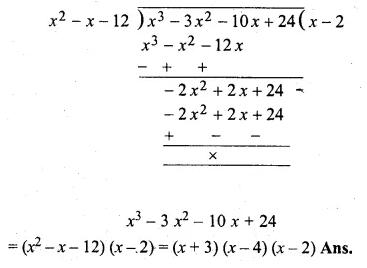Question 9.
If 2x3 + ax2 – 11x + b leaves remainder 0 and 42 when divided by (x – 2) and (x – 3) respectively, find the values of a and b. With these values of a and b, factorise the given expression.
Solution:
f(x) = 2x3 + ax2 – 11 x + b
Let x – 2 = 0, then x = 2,
Substituting the vaue of x in f(x)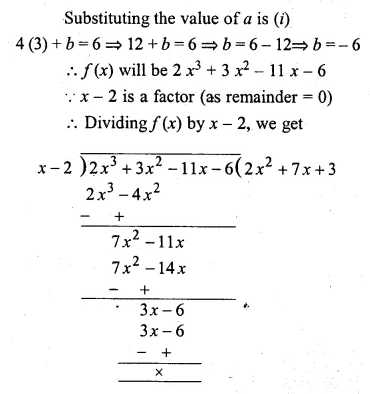Question 10.
If (2x + 1) is a factor of both the expressions 2x2 – 5x + p and 2x2 + 5x + q, find the value of p and q. Hence find the other factors of both the polynomials.
Solution:
Let 2x + 1 = 0, then 2x = – 1
x = $$– \frac { 1 }{ 2 }$$
Substituting the value of x in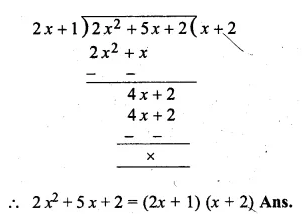Question 11.
When a polynomial f(x) is divided by (x – 1), the remainder is 5 and when it is,, divided by (x – 2), the remainder is 7. Find – the remainder when it is divided by (x – 1) (x – 2).
Solution:
When f(x) is divided by (x – 1),
Remainder = 5
Let r – 1 = 0 => x = 1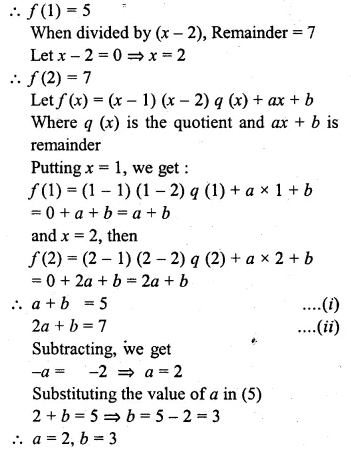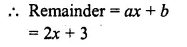Hope given ML Aggarwal Class 10 Solutions for ICSE Maths Chapter 7 Factorization Chapter Test are helpful to complete your math homework.

If you have any doubts, please comment below. APlusTopper try to provide online math tutoring for you.